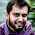17.9.15

calculate total= 1*1*1 + 2*2*2 + 3*3*3 + 4*4*4 using c++

Given an  int variable  n that has been initialized to a positive value and, in addition,  int variables  k and  total that have already been declared, use a  do...while loop to compute the sum of the cubes of the first  n whole numbers, and store this value in  total . Thus if  n equals 4, your code should put 1*1*1 + 2*2*2 + 3*3*3 + 4*4*4 into  total . Use no variables other than  n ,  k , and  total .

Solution
total=0;
k=0;
do
{
total+=k*k*k;
k++;
}
while (k<=n);

1 comment:

1.ID #201611036